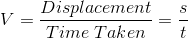# Unit of Velocity

## Introduction:

The velocity of an object is usually defined as the rate of displacement that a particle or an object undergoes within a span of time. Velocity is stated to be a physical vector quantity meaning both direction and magnitude have to be taken into consideration in order to define it.

However, speed and velocity should not be confused. There is a great distinction between the two, for example, velocity tells us about the speed of the object and in which direction it is moving while speed gives us an idea of how fast the object is moving. While it is important to remember this fact, we will study the unit of velocity in this article.

You may also want to check out these topics given below!

## What is the SI Unit of Velocity?

First, let us take a look at the formula of velocityHere,

• V is the velocity,
• s is displacement
• t is the time taken

If we remember, displacement is usually addressed in meters, and time taken is depicted in seconds. Therefore, velocity is expressed in meters/second or m/s.

The SI unit of velocity is meter per second (m/s).

Alternatively, the velocity magnitude can also be expressed in centimeters per second (cm/s).

### Some Other Velocity Units

Some of the other units that identify velocity are:

• Kilometers per hour
• Knot (unit)
• Feet per minute
• Foot per second
• Meter per hour
• Miles per hour
• Inch per second
• Speed of light

Stay tuned with BYJU’S for more such interesting articles. Also, register to “BYJU’S-The Learning App” for loads of interactive, engaging Physics-related videos and an unlimited academic assist.

Test your Knowledge on unit of velocity

#### 1 Comment

1. kaustubh

thanks it hepled me